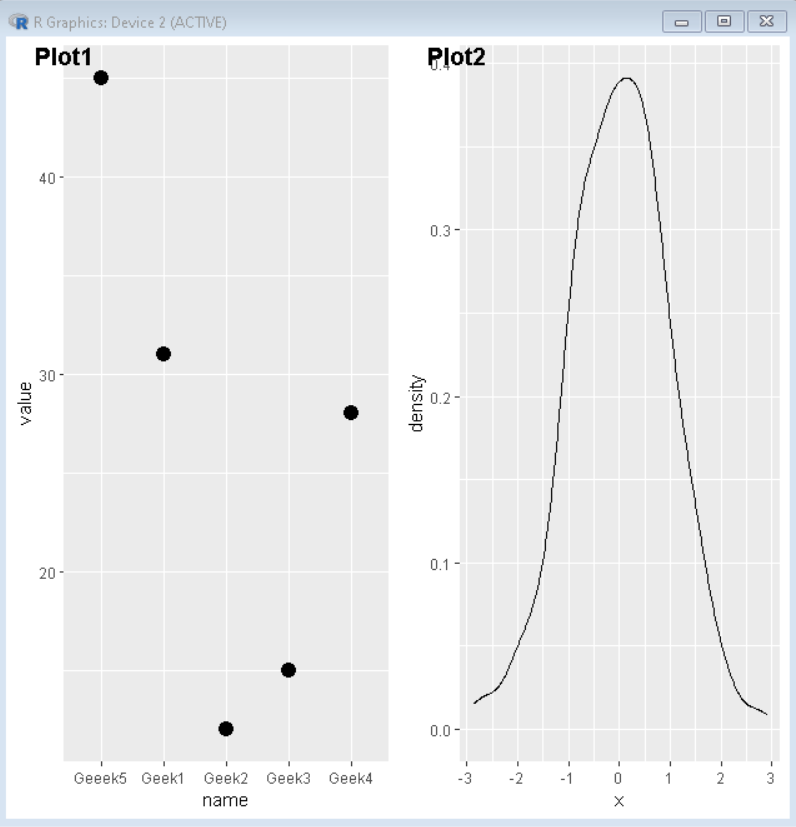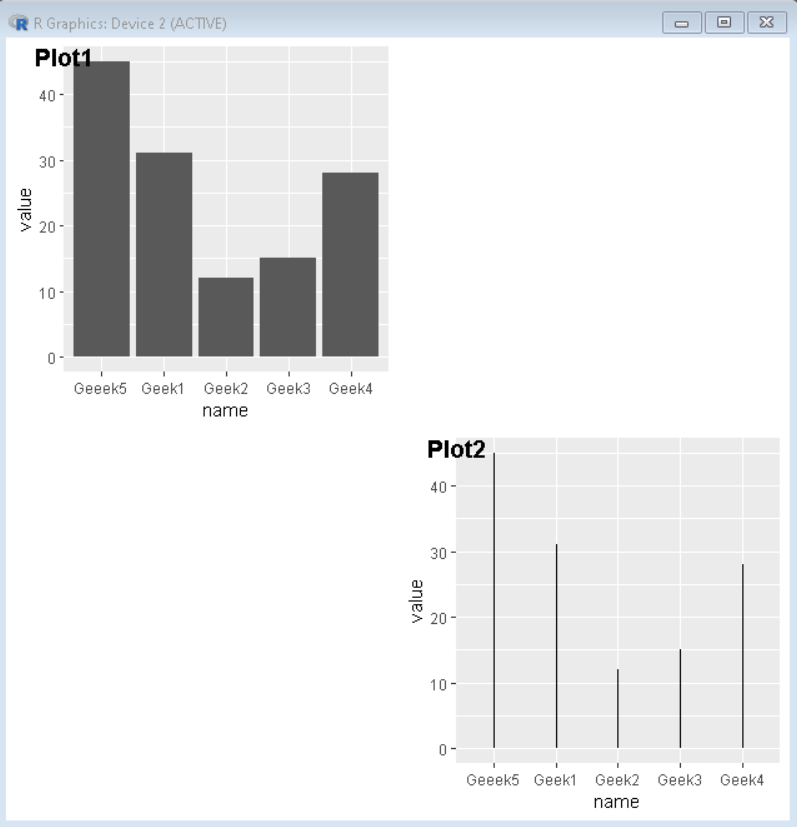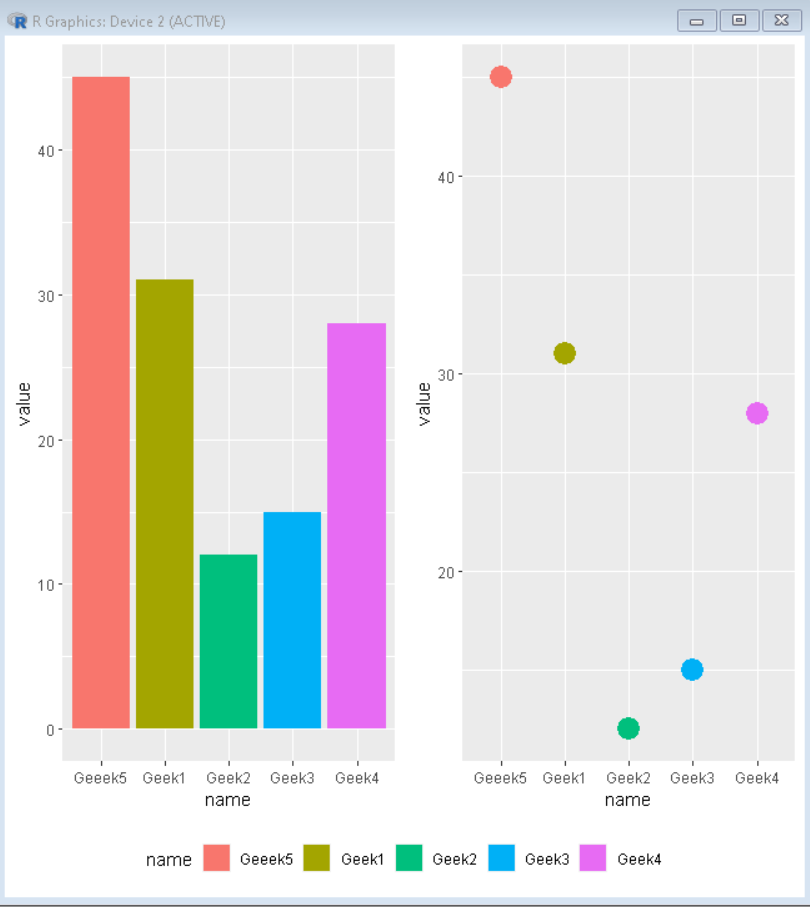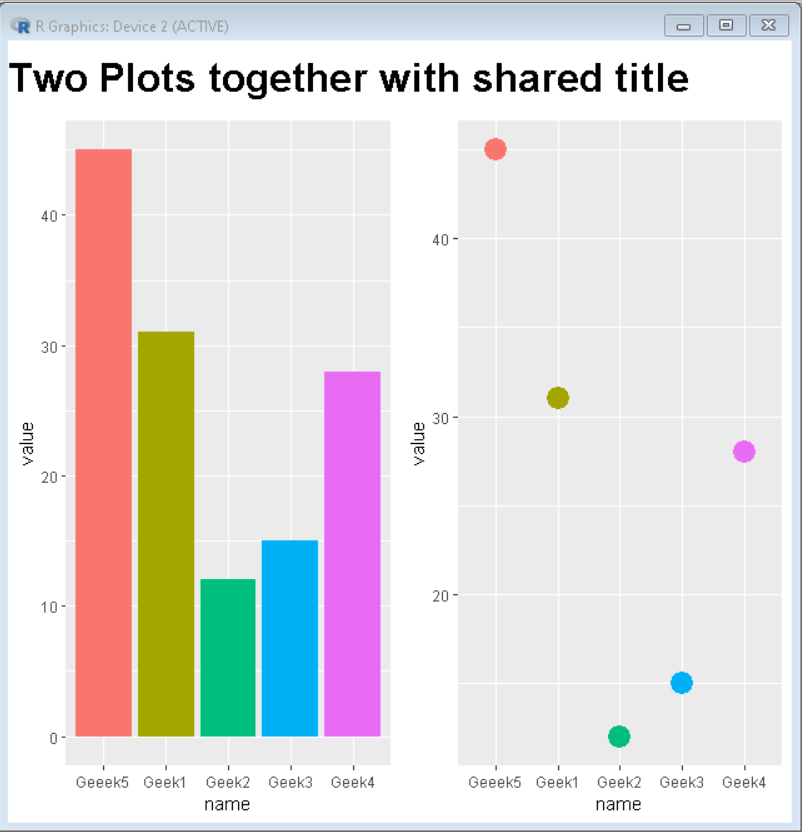Open in App
Not now

# How To Join Multiple ggplot2 Plots with cowplot?

• Last Updated : 17 Oct, 2021

In this article, we are going to see how to join multiple ggplot2 plots with cowplot. To join multiple ggplot2 plots, we use the plot_grid() function of the cowplot package of R Language.

Syntax: plot_grid(plot1,plot2,label=<label-vector>, ncol, nrow)

Parameters:

• plot1 and plot2 are plots that need to be joined.
• <label-vector> is a vector that has a collection of labels for plots.
• ncol is the number of columns canvas will be divided in.
• nrow is the number of rows canvas will be divided in.

## R

 `# Create sample data ` `set.seed``(5642)                              ` `sample_data1 <- ``data.frame``( ` `  ``name = ``c``(``"Geek1"``,``"Geek2"``,``"Geek3"``, ` `           ``"Geek4"``,``"Geeek5"``) , ` `  ``value=``c``(31,12,15,28,45)) ` ` `  `sample_data2 <- ``data.frame``(x = ``rnorm``(400)) ` ` `  `# Load ggplot2 and cowplot ` `library``(``"ggplot2"``)  ` `library``(``"cowplot"``) ` ` `  `# Create both plot and store in variable ` `plot1<-``ggplot``(sample_data1, ` `              ``aes``(x = name, y = value)) + ` `geom_point``(size=4) ` ` `  `plot2<-``ggplot``(sample_data2, ``aes``(x = x)) + ` `geom_density``(alpha=0.8) ` `plot_grid``(plot1, plot2, labels = ``c``(``'Plot1'``, ``'Plot2'``))`

Output:Output

### Example 2: Create a gap between plots using NULL as plot value.

To fix the number of columns we use ncol attribute of plot_grid() function.

## R

 `# Create sample data ` `set.seed``(5642)                              ` `sample_data <- ``data.frame``( ` `  ``name = ``c``(``"Geek1"``,``"Geek2"``,``"Geek3"``, ` `           ``"Geek4"``,``"Geeek5"``) , ` `  ``value = ``c``(31,12,15,28,45)) ` ` `  `# Load ggplot2 and cowplot ` `library``(``"ggplot2"``)  ` `library``(``"cowplot"``) ` ` `  `# Create both plot and store in variable ` `plot1<-``ggplot``(sample_data, ``aes``(x=name, y=value)) + ` `geom_bar``(stat = ``"identity"``) ` ` `  `plot2<-``ggplot``(sample_data, ``aes``(x = name, y=value)) + ` `geom_segment``( ``aes``(x=name, xend=name, y=0, yend=value))  ` ` `  `plot_grid``(plot1,``NULL``, ``NULL``, plot2, labels = ``c``( ` `  ``'Plot1'``,``''``,``''``, ``'Plot2'``), ncol=2)`

Output:Output

### Example 3: Side by Side plot with the shared legend.

To create a plot with a shared legend, we create plots without a legend and create a separate legend and combine them.

## R

 `# Create sample data ` `set.seed``(5642)                              ` `sample_data <- ``data.frame``(name=``c``(``"Geek1"``,``"Geek2"``,``"Geek3"``, ` `                                 ``"Geek4"``,``"Geeek5"``) , ` `                          ``value=``c``(31,12,15,28,45)) ` ` `  `# Load ggplot2 and cowplot ` `library``(``"ggplot2"``)  ` `library``(``"cowplot"``) ` ` `  `# Create both plot and store in variable without legend ` `plot1<-``ggplot``(sample_data, ``aes``(x=name, y=value, fill=name)) + ` `geom_bar``(stat = ``"identity"``) + ` `theme``(legend.position = ``"none"``) ` ` `  `plot2<-``ggplot``(sample_data, ``aes``(x = name, y=value, fill=name)) +   ` `geom_point``(``aes``(colour = ``factor``(name)), size = 6)+ ` `theme``(legend.position = ``"none"``) ` ` `  `# combine both plot using plot_grid() ` `combined_plot<-``plot_grid``(plot1, plot2,ncol=2) ` ` `  `# extract legend from plot2 ` `legend <- ``get_legend``( ` `  ``plot1 + ` `    ``guides``(color = ``guide_legend``(nrow = 1)) + ` `    ``theme``(legend.position = ``"bottom"``) ` `) ` ` `  `# Combine combined plot and legend using plot_grid() ` `plot_grid``(combined_plot, legend,ncol=1,rel_heights = ``c``(1, .1))`

Output:Output

### Example 4: Side by Side plot with a shared title

To create a plot with a shared title, we create plots without titles and create a separate title and combine them.

## R

 `# Create sample data ` `set.seed``(5642)                              ` `sample_data <- ``data.frame``(name=``c``(``"Geek1"``,``"Geek2"``, ` `                                 ``"Geek3"``,``"Geek4"``,``"Geeek5"``) , ` `                          ``value=``c``(31,12,15,28,45)) ` ` `  `# Load ggplot2 and cowplot ` `library``(``"ggplot2"``)  ` `library``(``"cowplot"``) ` ` `  `# Create both plot and store in variable without legend ` `plot1<-``ggplot``(sample_data, ``aes``(x=name, y=value, fill=name)) + ` `geom_bar``(stat = ``"identity"``) + ` `theme``(legend.position = ``"none"``) ` ` `  `plot2<-``ggplot``(sample_data, ``aes``(x = name, y=value, fill=name)) +   ` `geom_point``(``aes``(colour = ``factor``(name)), size = 6)+ ` `theme``(legend.position = ``"none"``) ` ` `  `# combine both plot using plot_grid() ` `combined_plot<-``plot_grid``(plot1, plot2,ncol=2) ` ` `  `# create title for plot ` `title <- ``ggdraw``() +  ` `  ``draw_label``( ` `    ``"Two Plots together with shared title"``, ` `    ``fontface = ``'bold'``, ` `    ``x = 0, ` `    ``hjust = 0, ` `    ``size = 24, ` `  ``) ` `# Combine combined plot and title using plot_grid() ` `plot_grid``(title, combined_plot ,ncol=1,rel_heights = ``c``(0.1, 1))`

Output:Output

My Personal Notes arrow_drop_up
Related Articles